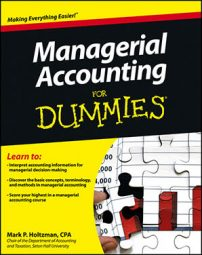##### Managerial Accounting For DummiesNet present value techniques use time value of money tools to estimate the current value of a series of future cash flows. Consider a company that has \$100 right now, on which it can earn 12-percent interest:

PV = \$100
i =0.12

To determine the future value of this investment after one year, just multiply the present value by one plus the interest rate:

PV(1 + i) = FV
100(1 + 0.12) = FV
100 x 1.12 = FV
\$112 = FV

This formula works in both directions. Suppose that you know that you need \$500 one year from now, and the expected interest rate is 11 percent. To figure out the present value, plug the \$500 future value into the formula:

PV(1 + i) = FV
PV(1 + 0.11) = 500
PV = 500 / 1.11
PV = \$450.45

In this case, if you start with \$450.45 today and put it away to earn 11-percent interest for one year, you’ll have \$500 one year from now.

To simplify the math in time value of money problems, and to avoid having to memorize long formulas, focus on the interest factor of “one plus the interest rate,” or (1 + i). Use this factor to convert back and forth between present and future values: To get the future value, multiply this factor by the present value. If you need the present value, divide the future value by this factor.

For example, if the interest rate is 12 percent, you focus on the interest rate factor, which equals 1.12, or (1 + 0.12). If present value were equal to \$100, the future value would equal \$100 x 1.12, or \$112. Working in the opposite direction, the present value of \$112 is just \$112 ÷ 1.12, or \$100.